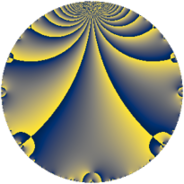# Properties

 Label 1850.2.a.bLevel $1850$ Weight $2$ Character orbit 1850.a Self dual yes Analytic conductor $14.772$ Analytic rank $1$ Dimension $1$ CM no Inner twists $1$

# Related objects

Show commands: Magma / PariGP / SageMath

## Newspace parameters

comment: Compute space of new eigenforms

[N,k,chi] = [1850,2,Mod(1,1850)]

mf = mfinit([N,k,chi],0)

lf = mfeigenbasis(mf)

from sage.modular.dirichlet import DirichletCharacter

H = DirichletGroup(1850, base_ring=CyclotomicField(2))

chi = DirichletCharacter(H, H._module([0, 0]))

N = Newforms(chi, 2, names="a")

//Please install CHIMP (https://github.com/edgarcosta/CHIMP) if you want to run this code

chi := DirichletCharacter("1850.1");

S:= CuspForms(chi, 2);

N := Newforms(S);

 Level: $$N$$ $$=$$ $$1850 = 2 \cdot 5^{2} \cdot 37$$ Weight: $$k$$ $$=$$ $$2$$ Character orbit: $$[\chi]$$ $$=$$ 1850.a (trivial)

## Newform invariants

comment: select newform

sage: f = N # Warning: the index may be different

gp: f = lf \\ Warning: the index may be different

 Self dual: yes Analytic conductor: $$14.7723243739$$ Analytic rank: $$1$$ Dimension: $$1$$ Coefficient field: $$\mathbb{Q}$$ Coefficient ring: $$\mathbb{Z}$$ Coefficient ring index: $$1$$ Twist minimal: yes Fricke sign: $$1$$ Sato-Tate group: $\mathrm{SU}(2)$

## $q$-expansion

comment: q-expansion

sage: f.q_expansion() # note that sage often uses an isomorphic number field

gp: mfcoefs(f, 20)

 $$f(q)$$ $$=$$ $$q - q^{2} - 2 q^{3} + q^{4} + 2 q^{6} - q^{8} + q^{9}+O(q^{10})$$ q - q^2 - 2 * q^3 + q^4 + 2 * q^6 - q^8 + q^9 $$q - q^{2} - 2 q^{3} + q^{4} + 2 q^{6} - q^{8} + q^{9} + 4 q^{11} - 2 q^{12} + 2 q^{13} + q^{16} - 8 q^{17} - q^{18} - 5 q^{19} - 4 q^{22} - q^{23} + 2 q^{24} - 2 q^{26} + 4 q^{27} + 10 q^{29} - 4 q^{31} - q^{32} - 8 q^{33} + 8 q^{34} + q^{36} - q^{37} + 5 q^{38} - 4 q^{39} + 7 q^{41} + 9 q^{43} + 4 q^{44} + q^{46} - 6 q^{47} - 2 q^{48} - 7 q^{49} + 16 q^{51} + 2 q^{52} + 3 q^{53} - 4 q^{54} + 10 q^{57} - 10 q^{58} - 11 q^{59} + 2 q^{61} + 4 q^{62} + q^{64} + 8 q^{66} - 2 q^{67} - 8 q^{68} + 2 q^{69} + 14 q^{71} - q^{72} - 3 q^{73} + q^{74} - 5 q^{76} + 4 q^{78} - 11 q^{79} - 11 q^{81} - 7 q^{82} - 8 q^{83} - 9 q^{86} - 20 q^{87} - 4 q^{88} - 2 q^{89} - q^{92} + 8 q^{93} + 6 q^{94} + 2 q^{96} + 8 q^{97} + 7 q^{98} + 4 q^{99}+O(q^{100})$$ q - q^2 - 2 * q^3 + q^4 + 2 * q^6 - q^8 + q^9 + 4 * q^11 - 2 * q^12 + 2 * q^13 + q^16 - 8 * q^17 - q^18 - 5 * q^19 - 4 * q^22 - q^23 + 2 * q^24 - 2 * q^26 + 4 * q^27 + 10 * q^29 - 4 * q^31 - q^32 - 8 * q^33 + 8 * q^34 + q^36 - q^37 + 5 * q^38 - 4 * q^39 + 7 * q^41 + 9 * q^43 + 4 * q^44 + q^46 - 6 * q^47 - 2 * q^48 - 7 * q^49 + 16 * q^51 + 2 * q^52 + 3 * q^53 - 4 * q^54 + 10 * q^57 - 10 * q^58 - 11 * q^59 + 2 * q^61 + 4 * q^62 + q^64 + 8 * q^66 - 2 * q^67 - 8 * q^68 + 2 * q^69 + 14 * q^71 - q^72 - 3 * q^73 + q^74 - 5 * q^76 + 4 * q^78 - 11 * q^79 - 11 * q^81 - 7 * q^82 - 8 * q^83 - 9 * q^86 - 20 * q^87 - 4 * q^88 - 2 * q^89 - q^92 + 8 * q^93 + 6 * q^94 + 2 * q^96 + 8 * q^97 + 7 * q^98 + 4 * q^99

## Embeddings

For each embedding $$\iota_m$$ of the coefficient field, the values $$\iota_m(a_n)$$ are shown below.

For more information on an embedded modular form you can click on its label.

comment: embeddings in the coefficient field

gp: mfembed(f)

Label $$\iota_m(\nu)$$ $$a_{2}$$ $$a_{3}$$ $$a_{4}$$ $$a_{5}$$ $$a_{6}$$ $$a_{7}$$ $$a_{8}$$ $$a_{9}$$ $$a_{10}$$
1.1
 0
−1.00000 −2.00000 1.00000 0 2.00000 0 −1.00000 1.00000 0
 $$n$$: e.g. 2-40 or 990-1000 Significant digits: Format: Complex embeddings Normalized embeddings Satake parameters Satake angles

## Atkin-Lehner signs

$$p$$ Sign
$$2$$ $$1$$
$$5$$ $$1$$
$$37$$ $$1$$

## Inner twists

This newform does not admit any (nontrivial) inner twists.

## Twists

By twisting character orbit
Char Parity Ord Mult Type Twist Min Dim
1.a even 1 1 trivial 1850.2.a.b 1
5.b even 2 1 1850.2.a.n yes 1
5.c odd 4 2 1850.2.b.h 2

By twisted newform orbit
Twist Min Dim Char Parity Ord Mult Type
1850.2.a.b 1 1.a even 1 1 trivial
1850.2.a.n yes 1 5.b even 2 1
1850.2.b.h 2 5.c odd 4 2

## Hecke kernels

This newform subspace can be constructed as the intersection of the kernels of the following linear operators acting on $$S_{2}^{\mathrm{new}}(\Gamma_0(1850))$$:

 $$T_{3} + 2$$ T3 + 2 $$T_{7}$$ T7

## Hecke characteristic polynomials

$p$ $F_p(T)$
$2$ $$T + 1$$
$3$ $$T + 2$$
$5$ $$T$$
$7$ $$T$$
$11$ $$T - 4$$
$13$ $$T - 2$$
$17$ $$T + 8$$
$19$ $$T + 5$$
$23$ $$T + 1$$
$29$ $$T - 10$$
$31$ $$T + 4$$
$37$ $$T + 1$$
$41$ $$T - 7$$
$43$ $$T - 9$$
$47$ $$T + 6$$
$53$ $$T - 3$$
$59$ $$T + 11$$
$61$ $$T - 2$$
$67$ $$T + 2$$
$71$ $$T - 14$$
$73$ $$T + 3$$
$79$ $$T + 11$$
$83$ $$T + 8$$
$89$ $$T + 2$$
$97$ $$T - 8$$